# SIMPLE INTEREST AND COMPOUND INTEREST-LEARN SERIES

Hi Bankersdaily Aspirants,

Aspirants,there are so many Neophyte who are going to attempt the IBPS Exam First Time.Here in the learn series we are updating the shortcut method of each topic section wise.Just Click on the below button to view the  Shortcut Method of each topic.

Aspirants SIMPLE INTEREST AND COMPOUND INTEREST topic is asked in IBPS Exams.The Questions from Simple Interest and Compound Interest is asked for 1 Marks in Prelims Exam and 2 Marks in Mains Exam.

SIMPLE INTEREST:

If the interest on a sum borrowed for certain period is reckoned uniformly, then it is called simple interest.

Let Principal = P,

Rate = R% per annum (p.a.) and

Time = T years. Then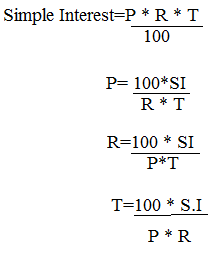Amount=Principal + SI

### CALCULATING SI,WHEN P,R,T ARE GIVEN:

1.Find the Simple Interest when the Principal is Rs.10000 Rate is 10% and Time is 2years and 4months?

Explanation

P=Rs.10000

Rate=10%

Time=2yrs and 1/3 years=2(1/3)  =7/3 years.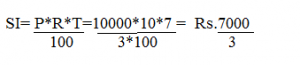#### 2.Find the Simple Interest when the Principal is Rs.10000 Rate of interest is 10% and Time is 2years and 73 days.

Explanation

Since it is not mentioned as leap year so we take it as ordinary year-365days

T=2 (73/365) =11/5 years

Principal=Rs.10000

Rate=10%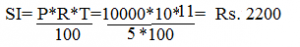### DIFFERENT RATE OF INTEREST FOR DIFFERENT YEARS:

1.What is the Simple Interest whose Principal is Rs.10000 and its Rate of interest for 5 years is separated as for First 2 year=10%   next year=20%  and last 2 year=25%?

Explanation

S.I.=P[(R1*T1)+(R2*T2)+(R3*T3)]/100

=10000[(2*10)+(1*20)+(2*25)]/100

=10000[20+20+50]/100

=10000/100

=Rs.9000

### CHANGE IN INTEREST:

1.A man invests Principal of Rs.10000 for 2 years.What is the change in SI when rate of interest changes from 10% to 15%?

Explanation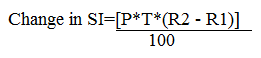=10000*2*5/100

=Rs.1000

Change in SI when two time Period is given is calculated by=P*R*(T1-T2)/100

Change in SI when two Principals are given = R*T*(P1-P2)/100

### BASED ON EFFICIENCY: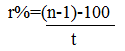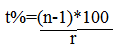where n=number of times and t=time in yrs

1.A sum becomes 5 times in 20 years at SI.Find the rate of interest?

Explanation

ORDINARY METHOD: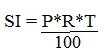SI=5P-P=4P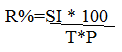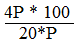=400/20   =20%

ALTERNATE METHOD: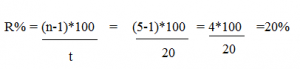### EQUALITY OF INTEREST:

1.If a sum is invested by A at 20% per annum for 2years ,B  at  10% per annum for 3 years  and C at 5% per annum for 4years.If SI received from all of three persons are equal. Find the ratio A,B,C?

Explanation

ORDINARY METHOD:

Formula for Simple Interest: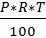Ration for A,B and C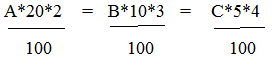40A:30B:20C=1

4A=3B=2C=1

A:B:C =1/4 :1/3 :1/2

A:B:C=3:4:6

ALTERNATE METHOD: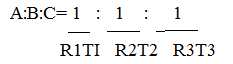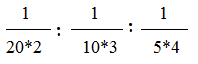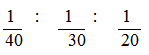=15:20:30

=3:4:6

For finding amount when SI are equal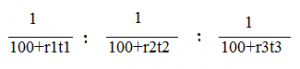### PARTIAL PRINCIPAL:

1.A sum of Rs.20000 is distributed in 2 schemes namely A at 10%per annum  and   B at 15% per annum at the end of 2 years he gets Rs.5200.What is the amount invested in scheme B?

Explanation

ORDINARY METHOD:

Let the money invested in scheme A=x, the money invested in scheme B=20000-x.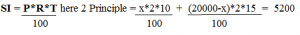A=X=8000   B=12000

ALTERNATE METHOD:

BY ALLEGATION:

Principal=20000 SI=5200 T=2years therefore R=13%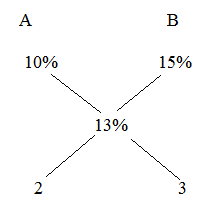20000* (3/5) =Rs.12000

The amount invested in scheme B=Rs.12000

### You May also like to Read Other Articles:

HOW TO SOLVE PROBLEMS ON PERMUTATIONS-LEARN SERIES

HOW TO SOLVE PROBLEMS ON AGES -PART 2

DIRECT INEQUALITIES “EITHER OR” CASES PART III